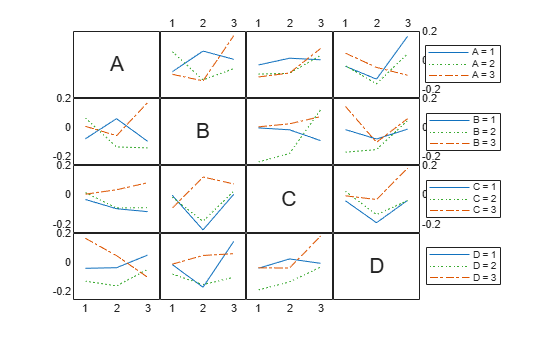Main Content

# interactionplot

Interaction plot for grouped data

## Syntax

```interactionplot(Y,GROUP) interactionplot(Y,GROUP,'varnames',VARNAMES) [h,AX,bigax] = interactionplot(...) ```

## Description

`interactionplot(Y,GROUP)` displays the two-factor interaction plot for the group means of matrix `Y` with groups defined by entries in `GROUP`, which can be a cell array or a matrix. `Y` is a numeric matrix or vector. If `Y` is a matrix, the rows represent different observations and the columns represent replications of each observation. If `Y` is a vector, the rows give the means of each entry in `GROUP`. If `GROUP` is a cell array, then each cell of `GROUP` must contain a grouping variable that is a categorical variable, numeric vector, character matrix, string array, or single-column cell array of character vectors. If `GROUP` is a matrix, then its columns represent different grouping variables. Each grouping variable must have the same number of rows as `Y`. The number of grouping variables must be greater than 1.

The interaction plot is a matrix plot, with the number of rows and columns both equal to the number of grouping variables. The grouping variable names are printed on the diagonal of the plot matrix. The plot at off-diagonal position (i,j) is the interaction of the two variables whose names are given at row diagonal (i,i) and column diagonal (j,j), respectively.

`interactionplot(Y,GROUP,'varnames',VARNAMES)` displays the interaction plot with user-specified grouping variable names `VARNAMES`. `VARNAMES` is a character matrix, a string array, or a cell array of character vectors, one per grouping variable. Default names are `'X1'`, `'X2'`, ... .

`[h,AX,bigax] = interactionplot(...)` returns a handle `h` to the figure window, a matrix `AX` of handles to the subplot axes, and a handle `bigax` to the big (invisible) axes framing the subplots.

## Examples

collapse all

Randomly generate data for a response variable `y` .

```rng default; % For reproducibility y = randn(1000,1);```

Randomly generate data for four three-level factors.

`group = ceil(3*rand(1000,4));`

Display the interaction plots for the factors and name the factors 'A', 'B', 'C', 'D'.

`interactionplot(y,group,'varnames',{'A','B','C','D'})`## See Also

Introduced in R2006b

Download ebook